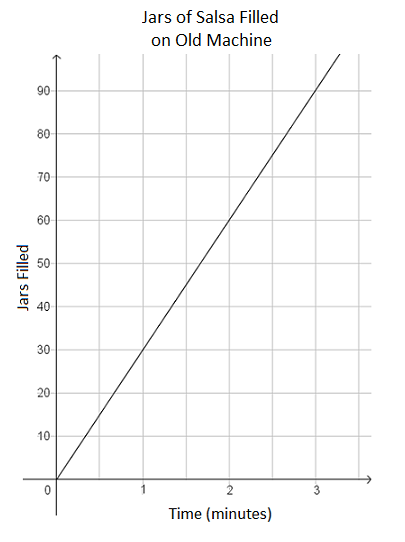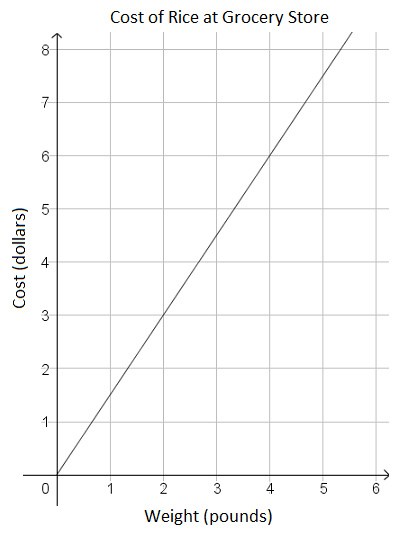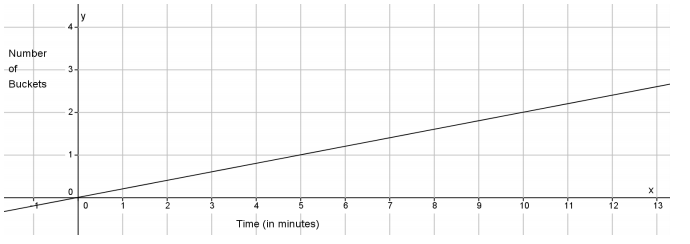# Linear Relationships

## Objective

Compare proportional relationships represented in different ways.

## Common Core Standards

### Core Standards

?

• 8.EE.B.5 — Graph proportional relationships, interpreting the unit rate as the slope of the graph. Compare two different proportional relationships represented in different ways. For example, compare a distance-time graph to a distance-time equation to determine which of two moving objects has greater speed.

?

• 7.RP.A.2

## Criteria for Success

?

1. Compare graphs of proportional relationships to other situations represented as tables, equations, and verbal descriptions.
2. Determine the rate of change or unit rate in equations, tables, and verbal descriptions.
3. Solve problems using proportional reasoning.

## Tips for Teachers

?

Lessons 3 and 4 address comparing proportional relationships. In this lesson, students compare across multiple forms of proportional relationships as they consider various applications.

#### Remote Learning Guidance

If you need to adapt or shorten this lesson for remote learning, we suggest prioritizing Choose two Anchor Problems (all benefit from worked examples). Find more guidance on adapting our math curriculum for remote learning here.

#### Fishtank Plus

• Problem Set
• Student Handout Editor
• Vocabulary Package

## Anchor Problems

?

### Problem 1

At a factory, a machine fills jars with salsa. The manager of the factory is considering buying a new machine that will fill 78 jars of salsa every 3 minutes. To support his decision, he wants to compare the rate of the new machine to the rate of the old machine that is currently in the factory.

The graph below shows the number of jars of salsa filled over time with the old machine.The manager is about to fill an order of 765 jars of salsa. How long would it take to fill this order on each machine? Should the manager consider replacing the old machine with the new one? Explain.

### Problem 2

Kristina and her sister, Tracee, are painting rooms in their house. The graph below represents the rate at which Kristina paints, and the table below shows how many square feet Tracee painted for given amounts of time. Both sisters paint at a constant pace.#### References

EngageNY Mathematics Grade 8 Mathematics > Module 4 > Topic C > Lesson 22Example 4

Grade 8 Mathematics > Module 4 > Topic C > Lesson 22 of the New York State Common Core Mathematics Curriculum from EngageNY and Great Minds. © 2015 Great Minds. Licensed by EngageNY of the New York State Education Department under the CC BY-NC-SA 3.0 US license. Accessed Dec. 2, 2016, 5:15 p.m..

Modified by The Match Foundation, Inc.

### Problem 3

The corner market sells rice by the pound using the equation ${y=1.25x}$, where $y$ represents the total cost for $x$ number of pounds.

The local grocery store also sells rice by the pound. The relationship between cost and weight at the grocery story is shown in the graph below.## Problem Set

?The following resources include problems and activities aligned to the objective of the lesson that can be used to create your own problem set.

?

Water flows out of Pipe A at a constant rate. Pipe A can fill 3 buckets of the same size in 14 minutes.

The graph below represents the rate at which Pipe B can fill the same-sized buckets.1. Write a linear equation that represents the number of buckets, $y$, that Pipe A can fill in $x$ minutes.

#### References

EngageNY Mathematics Grade 8 Mathematics > Module 4 > Topic C > Lesson 22Exit Ticket

Grade 8 Mathematics > Module 4 > Topic C > Lesson 22 of the New York State Common Core Mathematics Curriculum from EngageNY and Great Minds. © 2015 Great Minds. Licensed by EngageNY of the New York State Education Department under the CC BY-NC-SA 3.0 US license. Accessed Dec. 2, 2016, 5:15 p.m..

Modified by The Match Foundation, Inc.

?